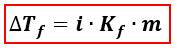Chemistry Practice Problems Freezing Point Depression Practice Problems Solution: A 5 g sample of CaI2 is completely dissolved in 22...

🤓 Based on our data, we think this question is relevant for Professor Weng's class at YU.

# Solution: A 5 g sample of CaI2 is completely dissolved in 22 g of water, what is the freezing point of the solution? Assume complete dissociation of the salt. Kf for water is 1.86°C/m.

###### Problem

A 5 g sample of CaI2 is completely dissolved in 22 g of water, what is the freezing point of the solution? Assume complete dissociation of the salt. Kf for water is 1.86°C/m.

###### Solution

We’re being asked to find the freezing point of a solution when CaI2 is dissolved in water. When calculating the freezing point of a solution, we’re going to use the equation for Freezing Point Depression.∆Tf = change in freezing point = Tf pure solvent –Tf solution
Kf = freezing point depression constant
i = van' t Hoff factor of the solute = no. of ions
m = molality

Let’s first calculate the molality of the solution.View Complete Written Solution

Freezing Point Depression

Freezing Point Depression

#### Q. What is the freezing point of an aqueous solution that boils at 106°C?       Kb = 0.51°C/m and Kf = 1.86°C/m. a. −4.55 °C b. −11.8 °C c. −21.9 °C ...

Solved • Tue Jul 25 2017 18:03:48 GMT-0400 (EDT)

Freezing Point Depression

#### Q. What is Kf for water if the freezing point was lowered to -12°C once 30 g of Sodium Chloride was added to 150 g of water?

Solved • Thu Jun 08 2017 16:42:11 GMT-0400 (EDT)

Freezing Point Depression

#### Q. Calculate the freezing temperature of a solution made by mixing 57.267 g of CaCl2 (MW = 110.98) in 350.00 g of water. k f = 1.86 C/mA. -2.74°CB. -11.0...

Solved • Wed Apr 26 2017 11:39:22 GMT-0400 (EDT)

Freezing Point Depression

#### Q. What mass of ethanol, C2H5OH a nonelectrolyte, must be added to 10.0L of water to give a solution that freezes at -10.0°C? Assume the density of water...

Solved • Mon Mar 06 2017 12:02:41 GMT-0500 (EST)